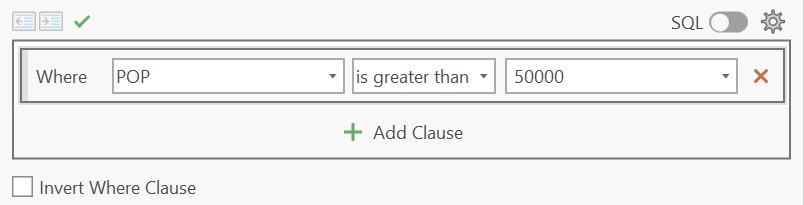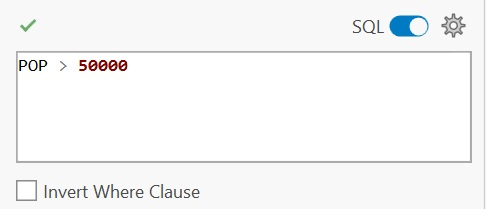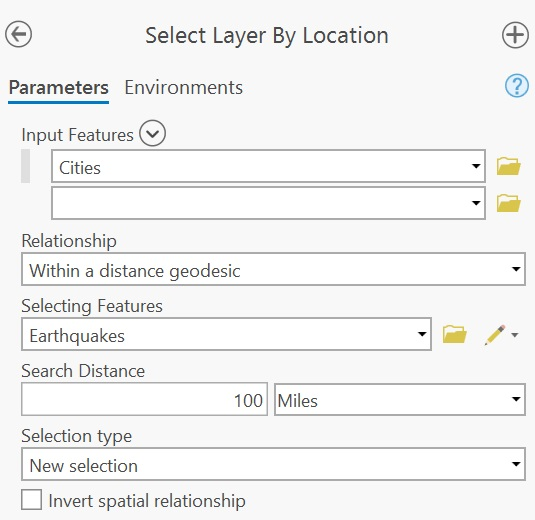# Chapter 8 Spatial Operations and Vector Overlays

## 8.1 Selection by Attribute

Features in a GIS layer can be selected graphically or by querying attribute values. For example, if a GIS layer represents land parcels, one could use the Area field to select all parcels having an area greater than 2.0 acres.

Set algebra is used to define conditions that are to be satisfied while Boolean algebra is used to combine a set of conditions.

### 8.1.1 Set Algebra

Set algebra consists of four basic operators:

• less than (<),
• greater than (>),
• equal to (=)
• not equal to (<>).

In some programming environments (such as R and Python), the equality condition is presented as two equal signs, ==, and not one. In such an environment x = 3 is interpreted as “pass the value 3 to x” and x == 3 is interpreted as "is x equal to 3?.

If you have a GIS layer of major cities and you want to identify all census tracts having a population count greater than 50000, you would write the expression as "POP" > 50000 (of course, this assumes that the attribute field name for population count is POP).Figure 8.1: An example of the Select Layer by Attributes tool in ArcGIS Pro where the pull-down menu is used to define the selection.Figure 8.2: An example of the Select Layer by Attributes tool in ArcGIS Pro where the SQL syntax is used to define the selection.Figure 8.3: Selected cities meeting the criterion are shown in cyan color in ArcGIS Pro.

The result of this operation is a selected subset of the Cities point layer. Note that in most GIS applications the selection process does not create a new layer.

### 8.1.2 Boolean Algebra

You can combine conditions from set algebra operations using the following Boolean algebra operators:

• or (two conditions can be met),
• and (two conditions must be met),
• not (condition must not be met).

Following up with the last example, let’s now select cities having a population greater than 50000 and that are in the US (and not Canada or Mexico). Assuming that the country field is labeled FIPS_CNTRY we could setup the expression as "POP" > 50000 AND "FIPS_CNTRY" = US. Note that a value need not be numeric. In this example we are asking that an attribute value equal a specific string value (i.e. that it equal the string 'US').Figure 8.4: Selected cities meeting POP > 50000 AND FIPS_CNTRY == US criteria are shown in cyan color.

## 8.2 Selection by location

We can also select features from one GIS layer based on their spatial association with another GIS layer. This type of spatial association can be broken down into four categories: adjacency (whether features from one layer share a boundary with features of another), containment (whether features from one layer are inside features of another), intersection (whether features of one layer intersect features of another), and distance (whether one feature is within a certain distance from another).

Continuing with our working example, we might be interested in Cities that are within 100 miles of earthquakes. The earthquake points are from another GIS layer called Earthquakes.Figure 8.5: An example of a Select Layer by Location tool in ArcGIS Pro. The spatial association chosen is distance.Figure 8.6: Selected cities meeting the criterion are shown in cyan color.

## 8.3 Vector Overlay

Map overlay refers to a group of procedures and techniques used in combining information from different data layers. This is an important capability of most GIS environments. Map overlays involve at least two input layers and result in at least one new output layer. A basic set of overlay tools include clipping, intersecting and unioning.

### 8.3.1 Clip

Clipping takes one GIS layer (the clip feature) and another GIS layer (the to-be-clipped input feature). The output is a clipped version of the original input layer. The output attributes table is a subset of the original attributes table where only records for the clipped polygons are preserved.Figure 8.7: The Maine counties polygon layer is clipped to the circle polygon. Note that the ouput layer is limited to the county polygon geometry and its attributes (and does not include the clipping circle polygon).

### 8.3.2 Intersect

Intersecting takes both layers as inputs then outputs the features from both layers that share the same spatial extent. Note that the output attribute table inherits attributes from both input layers (this differs from clipping where attributes from just one layer are carried through).Figure 8.8: The Maine counties polygon layer is intersected with the circle polygon. The ouput layer combines both intersecting geometries and attributes.

### 8.3.3 Union

Unioning overlays both input layers and outputs all features from the two layers. Features that overlap are intersected creating new polygons. This overlay usually produces more polygons than are present in both input layers combined. The output attributes table contains attribute values from both input features (note that only a subset of the output attributes table is shown in the following figure).Figure 8.9: The Maine counties polygon layer is unioned with the circle polygon. The ouput layer combines both (complete) geometries and attributes. Where spatial overlaps do not occur, most software will either assign a NULL value or a 0.